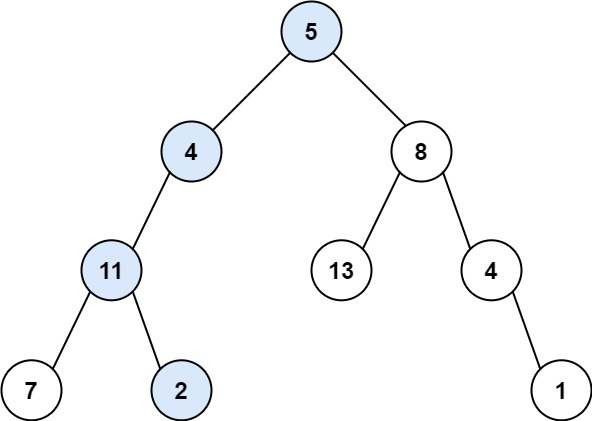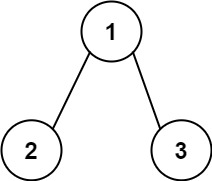# GeetCode Hub

Given the `root` of a binary tree and an integer `targetSum`, return `true` if the tree has a root-to-leaf path such that adding up all the values along the path equals `targetSum`.

A leaf is a node with no children.

Example 1:```Input: root = [5,4,8,11,null,13,4,7,2,null,null,null,1], targetSum = 22
Output: true
```

Example 2:```Input: root = [1,2,3], targetSum = 5
Output: false
```

Example 3:

```Input: root = [1,2], targetSum = 0
Output: false
```

Constraints:

• The number of nodes in the tree is in the range `[0, 5000]`.
• `-1000 <= Node.val <= 1000`
• `-1000 <= targetSum <= 1000`

/** * Definition for a binary tree node. * public class TreeNode { * int val; * TreeNode left; * TreeNode right; * TreeNode() {} * TreeNode(int val) { this.val = val; } * TreeNode(int val, TreeNode left, TreeNode right) { * this.val = val; * this.left = left; * this.right = right; * } * } */ class Solution { public boolean hasPathSum(TreeNode root, int targetSum) { } }